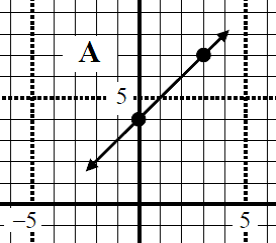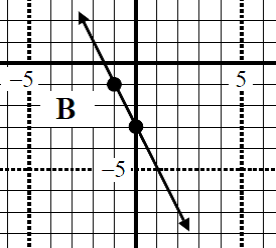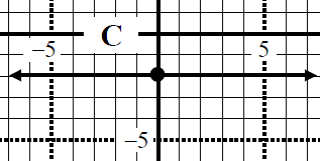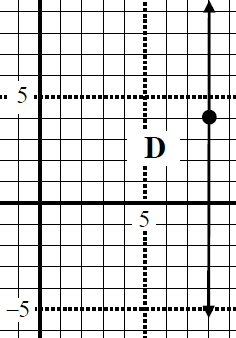# Parallel line equation#### Everything You Need in One Place

Homework problems? Exam preparation? Trying to grasp a concept or just brushing up the basics? Our extensive help & practice library have got you covered.#### Learn and Practice With Ease

Our proven video lessons ease you through problems quickly, and you get tonnes of friendly practice on questions that trip students up on tests and finals.#### Instant and Unlimited Help

Our personalized learning platform enables you to instantly find the exact walkthrough to your specific type of question. Activate unlimited help now!##### Examples
###### Lessons
1. Given the graph of linear equation, find the slope of parallel line equation.

1.2.3.4.2. The lines 2y - 6x - 4 = 0 and 6y - cx = 0 are parallel. Find "c".
1. Determine the equation of a line that is parallel to the line y = 4x - 1, and passes through the point (2, - 4). Answer in slope intercept form and general form.
1. Determine the equation of a line that is parallel to the line 3y - 9x - 3 = 0, and has the same x-int as the line 5y - 9x + 20 = 0. Answer in slope intercept form and general form.
###### Topic Notes
In this lesson, we will look at questions related to parallel line equation. We will try to determine parallel line equation with different given information such as, graphs, equations of other lines and points.